• ZOL首页
• 产品报价
• 论坛
• ZOL问答
• 更多
• 手机客户端

• 回答数

9

• 浏览数

1,297

### 9个回答 默认排序 默认排序 按时间排序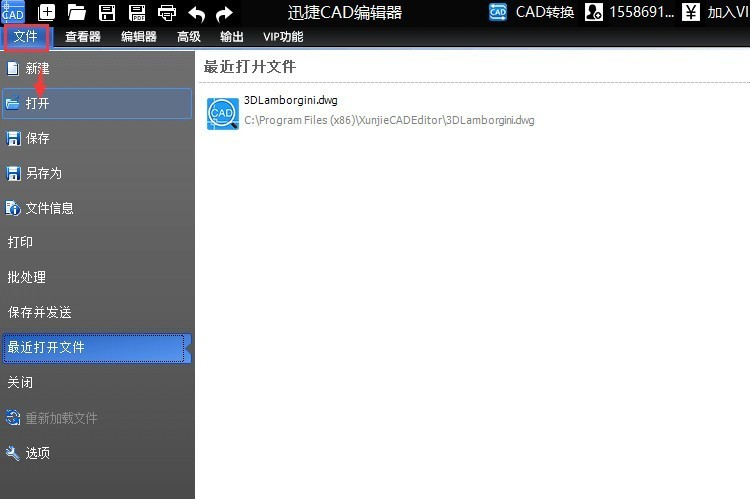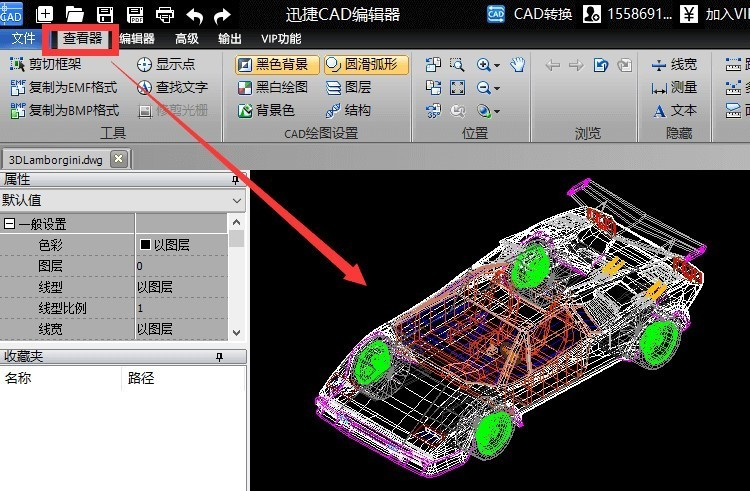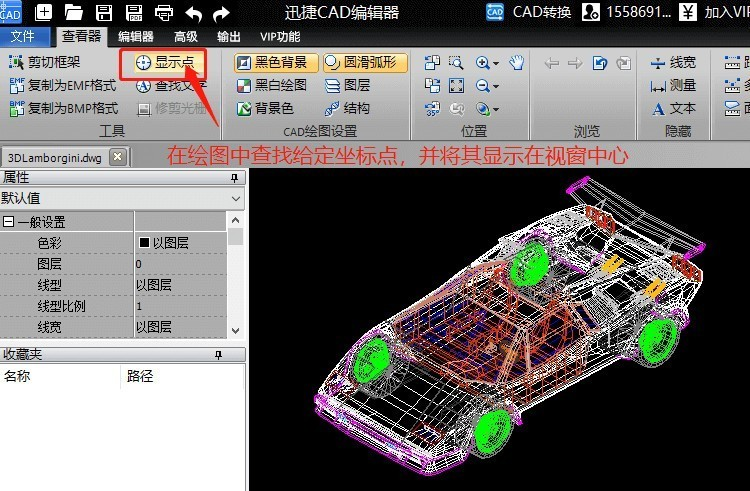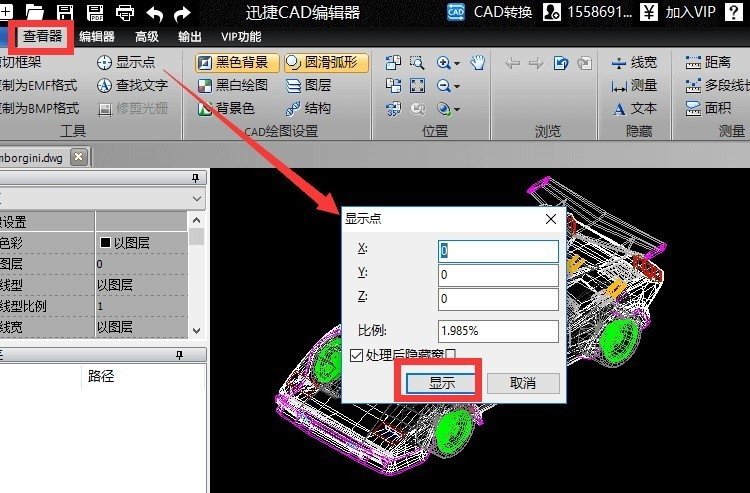[TH,R] = cart2pol(X,Y) TH 是极坐标的角度坐标，R 是距离坐标。
[TH,R,Z] = cart2pol(X,Y,Z) 这个函数同时支持3维直角坐标到柱坐标的转换。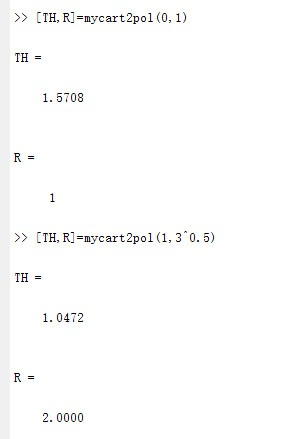function [ TH,R ] = mycart2pol(X,Y)
%this function transforms corresponding elements of data
%stored in Cartesian coordinates X,Y to polar coordinates
R=(X.^2+Y.^2).^0.5;
TH=atan(Y./X);
end### 举报成功